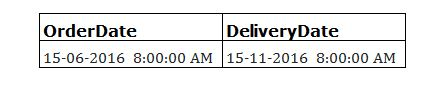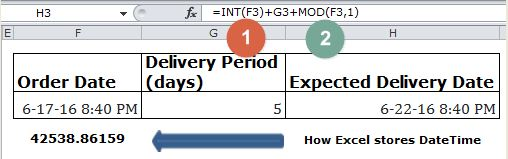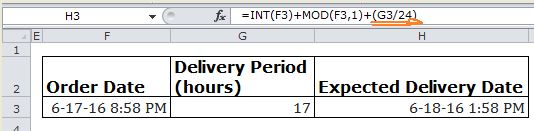#### Email Us

Follows

To update/extract the date or Time part separately in a `DateTime` Value is a lot easier Â if you understand thatÂ excel stores dates as sequential serial numbers and time as a fractional portion of a 24 hour day.

With the above understanding, you can combine `INT` and `MOD` functions to perform calculations on or extract the date or time portions.

For example if you have Order Dates and Delivery periods (days) Â you canÂ calculate expected Delivery date and Time## How it Works:

INT function extracts the Date portion of DateTime Value then adds the 5 days

`=INT(DateTime) + Period(days)`

MOD function extracts the Time portion which is the fractional part.

`=MOD(DateTime,1)`

What if the delivery period is in hours?NB: You need to convert the hours to fractional portion of a 24 hour for it to work thus

`=MOD(DateTime,1) + (hours/24)`

That’s All!

Give it a try with different days &/ Hours